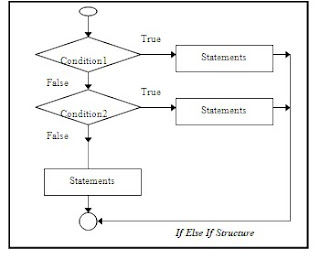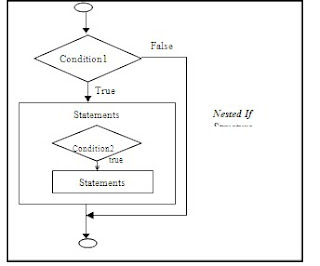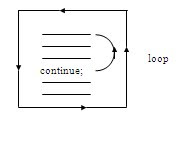/* related posts with thumb nails */

###Control Structure in Java:

Normally statements in a Java program are executed sequentially i.e. in the order in which they are written. This is called sequential execution. Transferring control to a desired location in a program is possible through Control structure. Java allows mainly kinds of control structures, which include:

1. Conditional control structure (Branching):

a) if

b) if else

c) if else if

d) nested if

e) switch

2. Jumping control structure:

a) break

b) Labelled break

c) continue

d) Labelled Continue

e) return

3. Iterative control structure (loops):

a) for loop

b) while loop

c) do while loop

d) for each (enhanced for) loop

1. Conditional control structure:

a) if:Syntax:

if (condition)

{

statements

}

Explanation:

If structure is also called as conditional statement. In If, statements get executed only when the condition is true. It omits the condition based statements when the condition is false. Braces are not necessary if only one statement is related to the condition.

Example:

if ( x > 0 )

System.out.println(“positive”);

b) if else:Syntax:

if (condition)

{

Statements

}

else

{

Statements

}

Explanation:

Statements in if block get executed only when the condition is true and statements in else block get executed only when the condition is false.

Example:

if ( x > y )

System.out.println( “x is big”);

else

System.out.println( “y is big or equal ”);

c) if else if:Syntax:

if (condition1)

{

Statements

}

else if (condition2)

{

Statements

}

else if (condition3)

{

Statements

}

.

.

.

else

{

statements

}

Explanation:

Statements get executed in loops only when the corresponding conditions are true. Statements in the final else block get executed when all other conditions are false. The control checks a condition only when all the afore-mentioned conditions are false.

Example:

if ( x > 0 )

System.out.println( “positive”);

else if ( x < 0)

System.out.println(“negative ”);

else

System.out.println( “zero”);

d) nested if:Syntax:

if (condition1)

{

if ( condition2)

{

Statements

}

}

Explanation:

Writing if in another if is nested if. Inner if is processed only when outer if’s condition is true. Hence, statements in inner-if get executed only when condition1 and condition2 are true.

Example:

if ( a > b )

{

if (a>c)

System.out.println( “ a is the biggest”);

}

e) switch:Syntax:

switch(variable)

{

case constant1: statment1

break;

case constant2: statement2

break;

.

.

default: statements

break;

}

Explanation:

switch is similar to if else if. Statement 1 gets executed when the variable is equal to constant1 and so on. Control comes to “default” portion when all the cases (constants), which have been mentioned do not match with the value of the variable. Here break and default are optional. When there is no break for a case it continues till it encounters break or the end of the switch loop.

Example:

k=x-y;

switch(k)

{

case 0: System.out.println( “ x and y are equal”);

break;

default: System.out.println( “ x and y are not equal”);

break;

}

2. Jumping control structure:

Explanation:

a) break:Break quits the control from corresponding iterative loop. Break is valid only in iterative loops and switch. Break is used to bring the control out of iterative loops intermediately.

Example:

for ( i=1 ; i<=10; i++)

{

System.out.println(“ hello”);

if (i==5)

break;

}

/* prints hello 5 times */

b) Labelled break:Explanation:

Labelled break quits the control from labeled iterative loop. The need of labelled break arises when we need to stop the execution of outer loop based on the condition written in inner loop. Labelled iterative loop is a normal iterative loop with a label given to it.

Example:

ABC:

for ( i=1 ; i<=10; i++)

{

System.out.println(“ hi”);

for (j=1 ; j<=10;j++)

{

System.out.println(“ hello”);

if (j==2)

break ABC;

}

}

Output:

hi

hello

hello

c) continue:Explanation:

continue moves the control the first statement in the corresponding iterative loop. “continue” is valid only in iterative loops. It omits the statements written after “continue” and restarts the iterative loop.

Example:

i=0;

while (true)

{

System.out.println(“ hello”);

if (i<5)

continue;

break;

}

/* prints hello 5 times */

d) Labelled Continue:Explanation:Labelled continue moves the control the first statement in the labelled iterative loop. It omits the statements written after “continue” and restarts the labeled iterative loop.

Example:

i=0;

XYZ:

while (true)

{

System.out.println(“ hi”);

while (true)

{

System.out.println(“ hello”);

if (i<2)

continue XYZ;

break XYZ;

}

}

Output

hi

hello

hi

hello

e) return:Explanation:

return is used in functions. It moves the control to calling function from the called module. "return” is also used to return values to the point where it is called. But it is possible to return only one value at a time.

Example:

int sum(int x, int y)

{

return x+y;

}

int k= sum(30,40);

Here “sum” function returns 70 into the variable k with the above call.

3. Iterative control structure (loops):

a) for loop:syntax:

for ( expression1 ; condition; expression2)

{

Statements

}

explanation:

Statements get executed as long as the condition is true. Generally expression1 includes initializing statements and expression2 includes statements that use increment, decrement, and assignment operators.

Example:

for( i=1 ; i<=10 ; i++)

System.out.println(“hello”);

/* prints hello 10 times */

b) while loop:

syntax:

while ( condition )

{

Statements

}Explanation:

Statements get executed as long as the condition is true. It checks the condition first and executes the statements later. It is also called entry control loop.

Example:

i=0;

while( i<10 )

{

System.out.println(“hello”);

i=i+1;

} /* prints hello 10 times */

c) do while loop:

Syntax:

do

{

Statements

}while(condition);Explanation:

Statements get executed as long as the condition is true. It is similar to while but, it executes the statements first and checks the condition later. It ensures at least one time execution. It is called exit control loop.

Example:

i=0;

do

{

System.out.println(“hello”);

i=i+1;

} while( i<10 );

/* prints hello 10 times */

d) for each (enhanced for) loop:

Syntax:

for (variable : expression)

{

Statements

}

Explanation:

The enhanced for loop is called as for each loop. It is introduced in J2SE 5.0. This is more used with arrays and enumerations. Generally to access the array elements, we use indexes. But, using for-each, we can directly have access to each element of the array without indexes.

Example:

int x[]= {10,20,30};

for (int k : x)

System.out.print(k+” “);

The above code prints: 10 20 30

Related Topics: